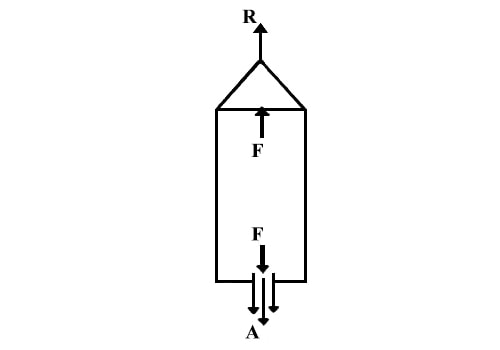# Principle of Rockets

A rocket is a vehicle which propels itself by ejecting a part of its mass. Rockets are used to carry the payloads (satellites). We have heard of the PSLV and GSLV rockets. All of them are based on Newton’s third law of motion.

Consider a hollow cylindrical vessel closed on both ends with a small hole at one end, containing a mixture of combustible fuels (Figure). If the fuel is ignited, it is converted into a gas under high pressure. This high pressure pushes the gas through the hole with an enormous force. This force represents the action A. Hence an opposite force, which is the reaction 12, will act on the vessel and make it move forward.

The force (Fm) on the escaping mass of gases and hence the rocket is proportional to the product of the mass of the gases discharged per unit time (dm/dt) and the velocity with which they are expelled (v).

Fm α (dm/dt) vFigure: Principle of Rockets

This force is known as momentum thrust. If the of pressure (Pe) of the escaping gases differs from the pressure (Po) in the region outside the rocket, there is an additional thrust called the velocity thrust (Fv) acts. It is given by Fv = A (Pe – Po) where A is the area of the nozzle through which the gases escape.

Hence, the total thrust on the rocket is F = Fm + Fv.